• matlab求协方差矩阵>> A=[0,0,0;2,0,2;]A =0 0 02 0 2>> v = diag(cov(A))'v =2 0 2help里面的cov 函数，你自己看一下吧!MATLAB怎么计算协方差>> x=rand(1,5);>> y=2*rand(1,5);>> ...
matlab求协方差矩阵>> A=[0,0,0;2,0,2;]A =0 0 02 0 2>> v = diag(cov(A))'v =2 0 2help里面的cov 函数，你自己看一下吧!MATLAB 怎么计算协方差>> x=rand(1,5);>> y=2*rand(1,5);>> cov(x,y) %计算协方差ans =0.1079   -0.0225-0.0225    0.6148协方差分析是建立在方差分析和回归分析基础之上的一种统计分析方法。 方差分析是从质量因子的角度探讨因素不同水平对实验指标影响的差异。一般说来，质量因子是可以人为控制的。 回归分析是从数量因子的角度出发，通过建立回归方程来研究实验指标与一个(或几个)因子之间的数量关系。但大多数情况下，数量因子是不可以人为加以控制的。为什么考虑风险时，与市场组合的协方差更重要?组合的方差是有协方差矩阵的和决定的假如有N个资产构成一个组合那么协方差有N的平方减N个而方差只有N个所以前者重要这个告诉我们 股票的收益率看的是它对投资组合的风险贡献率 而不是自己的波动- -说得比较抽象Excel、Matlab的算出的协方差矩阵为什么不同大概是因为两种用的公式不一样。 因为你不可能根据样本得到协方差矩阵， 而只能根据样本做一个估计， 既然是估计那么当然有多种公式都是可行的， 而不能说哪一种就一定错【本文标题和网址】股票的协方差矩阵-matlab求协方差矩阵：http://www.jjta.cn/pzzx/160113.html声明本站分享的文章旨在促进信息交流，不以盈利为目的，本文观点与本站立场无关，不承担任何责任。如有伤害到您的利益，请联系站长删除！
展开全文• X = [12.5 586;  24 754;  15.3 850;  18 667;  31.2 750];%X是初始矩阵 CovX=cov(X); CorrX=corr(X);
X = [12.5 586;
24 754;
15.3 850;
18 667;
31.2 750];%X是初始矩阵
CovX=cov(X);
CorrX=corr(X);

展开全文• Covariance 翻译为协方差，因此，MATLAB里面的函数cov也就是求协方差了。至于MATLAB语言里面的协方差函数cov的语法是什么样的以及怎么用的，我们稍后再说，这里首先介绍下协方差相关的基础知识点。 本文内容参考自...
Covariance 翻译为协方差，因此，MATLAB里面的函数cov也就是求协方差了。至于MATLAB语言里面的协方差函数cov的语法是什么样的以及怎么用的，我们稍后再说，这里首先介绍下协方差相关的基础知识点。

本文内容参考自MATLAB的帮助手册，有的时候不得不说，数据手册才是最好的教材，不仅对于MATLAB，这里提供的都是原滋原味的官方内容。例如我经常去了解一些MATLAB中的相关函数，命令等，都可以通过MATLAB的数据手册；如果我想了解一些IP核以及与之相关的知识，我可以查看Xilinx的官方数据手册，内容应有尽有，相比而言，如果我去借一些书籍去查看FPGA的IP核，不仅版本陈旧，而已也有可能翻译的有问题，让人一知半解。

废话就说到这里，下面正式开始介绍。

目录

基础知识

协方差（Covariance）：

协方差矩阵（ covariance matrix）：

矩阵的协方差：

方差：（这是赠送的）

MATLAB中的 cov

语法格式：

C = cov(A)

C = cov(A,B)

C = cov(___,w)

C = cov(___,nanflag)

示例

C = cov(A) 举例（矩阵的协方差）

cov(A,B) 举例之两个向量之间的协方差

cov(A,B) 举例之两个矩阵之间的协方差

Specify Normalization Weight

Covariance Excluding NaN

基础知识

协方差（Covariance）：

对于两个随机变量向量A和B，那二者之间的协方差定义为：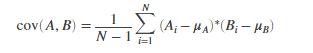其中表示向量A的均值，表示向量B的均值。

协方差矩阵（ covariance matrix）：

两个随机变量的协方差矩阵是每个变量之间成对协方差计算的矩阵，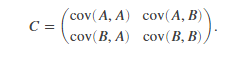矩阵的协方差：

对于矩阵A，其列各自是由观察组成的随机变量，协方差矩阵是每个列组合之间的成对协方差计算。 换一种说法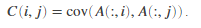方差：（这是赠送的）

对于由N个标量观测组成的随机变量向量A，方差定义为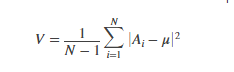其中u是A的均值：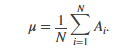一些方差定义使用归一化因子N而不是N-1，可以通过将w设置为1来指定。在任何一种情况下，假设均值具有通常的归一化因子N.

（注意：w是后面要说的MATLAB里面的协方差函数的一个参数而已，在具体的MATLAB函数里面可以通过设置w来指定归一化因子！）

MATLAB中的 cov

语法格式：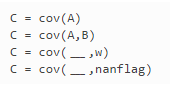下面逐个讲解：

C = cov(A)

C = cov(A) returns the covariance.

C = cov(A)返回协方差。

If A is a vector of observations, C is the scalar-valued variance.

如果A是一个观测向量，那么C是一个标量值的方差。

If A is a matrix whose columns represent random variables and whose rows represent observations, C is the covariance matrix with the corresponding column variances along the diagonal.

如果A是矩阵，其列表示随机变量，其行表示观测值，则C是协方差矩阵，沿对角线具有相应的列方差。（协方差矩阵的协方差是列的协方差值）

C is normalized by the number of observations-1. If there is only one observation, it is normalized by 1.

C由观察数-1归一化。 如果只有一个观察值，则将其标准化为1。

If A is a scalar, cov(A) returns 0. If A is an empty array, cov(A)returns NaN.

如果A是标量，则cov（A）返回0.如果A是空数组，则cov（A）返回NaN。

（你看看人家考虑的多周全！）

C = cov(A,B)

C = cov(A,B) returns the covariance between two random variables A and B.

C = cov(A,B) 返回两个随机变量A和B之间协方差。

If A and B are vectors of observations with equal length, cov(A,B) is the 2-by-2 covariance matrix.

如果A和B是同等长度的观测向量，那么C是一个2*2的协方差矩阵。

If A and B are matrices of observations, cov(A,B) treats A and B as vectors and is equivalent to cov(A(:),B(:)). A and B must have equal size.

如果A和B是观察矩阵，则cov（A，B）将A和B视为向量，并且等同于cov（A（:)，B（:)）。 A和B必须具有相同的大小。

If A and B are scalars, cov(A,B) returns a 2-by-2 block of zeros. If A and B are empty arrays, cov(A,B) returns a 2-by-2 block of NaN.

如果A和B是标量，则cov（A，B）返回2乘2的零块。 如果A和B是空数组，则cov（A，B）返回2乘2的NaN块。

C = cov(___,w)

C = cov(___,w) specifies the normalization weight for any of the previous syntaxes. When w = 0 (default), C is normalized by the number of observations-1. When w = 1, it is normalized by the number of observations.

C = cov（___，w）指定任何先前语法的归一化权重。 当w = 0（默认值）时，C由观测数-1归一化。 当w = 1时，它通过观察次数归一化。

C = cov(___,nanflag)

C = cov(___,nanflag) specifies a condition for omitting NaN values from the calculation for any of the previous syntaxes. For example, cov(A,'omitrows') will omit any rows of A with one or more NaN elements.

C = cov（___，nanflag）指定从任何先前语法的计算中省略NaN值的条件。 例如，cov（A，'omitrows'）将省略具有一个或多个NaN元素的A的任何行。

示例

下面举例说明重要的语法格式：

C = cov(A) 举例（矩阵的协方差）

Create a 3-by-4 matrix and compute its covariance

A = [5 0 3 7; 1 -5 7 3; 4 9 8 10];
C = cov(A)

C = 4×4

4.3333    8.8333   -3.0000    5.6667
8.8333   50.3333    6.5000   24.1667
-3.0000    6.5000    7.0000    1.0000
5.6667   24.1667    1.0000   12.3333



Since the number of columns of A is 4, the result is a 4-by-4 matrix.

由于矩阵A有4列，表示有4个随机变量，那么协方差矩阵是4*4的。

cov(A,B) 举例之两个向量之间的协方差

1. Create two vectors and compute their 2-by-2 covariance matrix.

A = [3 6 4];
B = [7 12 -9];
cov(A,B)

ans = 2×2

2.3333    6.8333
6.8333  120.3333

cov(A,B) 举例之两个矩阵之间的协方差

2. Create two matrices of the same size and compute their 2-by-2 covariance.

A = [2 0 -9; 3 4 1];
B = [5 2 6; -4 4 9];
cov(A,B)

ans = 2×2

22.1667   -6.9333
-6.9333   19.4667

这相当于求A(:)和B(:)的协方差，如下验证下：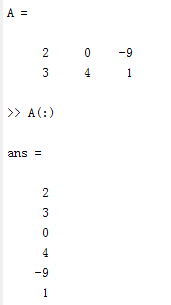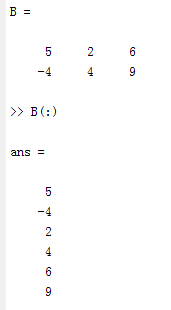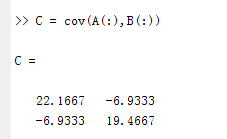对比下cov(A,B)发现是一致的：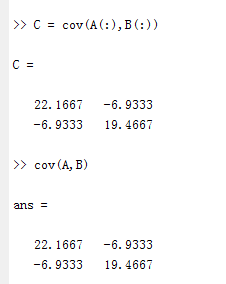Specify Normalization Weight

创建一个矩阵并计算由行数归一化的协方差。

A = [1 3 -7; 3 9 2; -5 4 6];
C = cov(A,1)

C = 3×3

11.5556    5.1111  -10.2222
5.1111    6.8889    5.2222
-10.2222    5.2222   29.5556

我觉得还是有必要比较下不归一化的情况：

>> A = [1 3 -7; 3 9 2; -5 4 6]

A =

1     3    -7
3     9     2
-5     4     6

>> C = cov(A,1)

C =

11.5556    5.1111  -10.2222
5.1111    6.8889    5.2222
-10.2222    5.2222   29.5556

>> C = cov(A)

C =

17.3333    7.6667  -15.3333
7.6667   10.3333    7.8333
-15.3333    7.8333   44.3333

Covariance Excluding NaN

创建矩阵并计算其协方差，排除包含NaN值的任何行。

A = [1.77 -0.005 3.98; NaN -2.95 NaN; 2.54 0.19 1.01]

A = 3×3

1.7700   -0.0050    3.9800
NaN   -2.9500       NaN
2.5400    0.1900    1.0100



C = cov(A,'omitrows')

C = 3×3

0.2964    0.0751   -1.1435
0.0751    0.0190   -0.2896
-1.1435   -0.2896    4.4104



展开全文• 一：matlab里面关于方差和协方差的几点需要...求协方差的函数：cov 1 如果a,b是向量，则： cov(a,b)相当于是[D(a),cov(a,b);cov(b,a),D(b)]，其中D(a)和D(b)分别代表了a,b的方差。cov(a,b)和cov(b,a)
一：matlab里面关于方差和协方差的几点需要注意的
求均值的函数：mean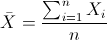求方差的函数：var
标准差：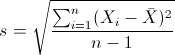方差：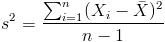求协方差的函数：cov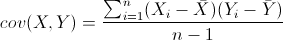1 如果a,b是向量，则：
cov(a,b)相当于是[D(a),cov(a,b);cov(b,a),D(b)]，其中D(a)和D(b)分别代表了a,b的方差。cov(a,b)和cov(b,a)分别代表了两者之间的协方差

举例：
>> a=[1.1 2.2 3 4.5 0.8]

a =

1.1000    2.2000    3.0000    4.5000    0.8000

>> b=[1.3 2.4 2.1 5 3.3]

b =

1.3000    2.4000    2.1000    5.0000    3.3000

>> cov(a,b)

ans =

2.2570    1.3595
1.3595    1.9970其中，对角线的值是两个向量的方差，斜对角线的是两个向量之间的协方差

2 如果a,b是矩阵，则：
cov(a,b)相当于是cov(a(:),b(:))，举例说明：
>> a=randn(4);
>> a

a =

-0.4326   -1.1465    0.3273   -0.5883
-1.6656    1.1909    0.1746    2.1832
0.1253    1.1892   -0.1867   -0.1364
0.2877   -0.0376    0.7258    0.1139
>> b=randn(4);
>> b

b =

1.0668    0.2944   -0.6918   -1.4410
0.0593   -1.3362    0.8580    0.5711
-0.0956    0.7143    1.2540   -0.3999
-0.8323    1.6236   -1.5937    0.6900
>> cov(a,b)

ans =

0.8513   -0.0860
-0.0860    1.0129

/
>> a(:)

ans =

-0.4326
-1.6656
0.1253
0.2877
-1.1465
1.1909
1.1892
-0.0376
0.3273
0.1746
-0.1867
0.7258
-0.5883
2.1832
-0.1364
0.1139

>> b(:)

ans =

1.0668
0.0593
-0.0956
-0.8323
0.2944
-1.3362
0.7143
1.6236
-0.6918
0.8580
1.2540
-1.5937
-1.4410
0.5711
-0.3999
0.6900

>> cov(a(:),b(:))

ans =

0.8513   -0.0860
-0.0860    1.0129

另外，matlab里面关于cov函数的说明解释的很详细：

cov(x), if x is a vector, returns the variance of x. For matrix input X, where each row is an observation, and each column is a variable, cov(X) is the covariance matrix. diag(cov(X)) is a vector of variances
for each column, and sqrt(diag(cov(X))) is a vector of standard deviations. cov(X,Y), where X and Y are matrices with the same number of elements, is equivalent to cov([X(:) Y(:)]).

cov(x) or cov(x,y) normalizes by N – 1, if N > 1, where N is the number of observations. This makes cov(X) the best unbiased estimate of the covariance matrix if the observations are from a normal distribution.
For N = 1, cov normalizes by N.

cov(x,1) or cov(x,y,1) normalizes by N and produces the second moment matrix of the observations about their mean. cov(X,Y,0) is the same as cov(X,Y) and cov(X,0) is the same as cov(X).

其使用示例可见：http://www.mathworks.cn/cn/help/matlab/ref/cov.html?searchHighlight=cov

参考：

http://pinkyjie.com/2010/08/31/covariance/

http://www.cnblogs.com/cvlabs/archive/2010/05/08/1730319.html
http://blog.csdn.net/faceRec/article/details/1697362
http://blog.csdn.net/ybdesire/article/details/6270328
展开全文• 介绍了Matlab 中如何使用 mean 、var 、std、cov 函数等
• 共回答了23个问题采纳率：95.7%Matlab函数：mean>>X=[1,2,3]>>mean(X)=2如果X是一个矩阵,则其均值是一个向量组.mean(X,1)为列向量的均值,mean(X,2)为行向量的均值.>>X=[1 2 34 5 6]>>mean(X...
• 本博文源于matlab基础，主要对协方差矩阵如何生成进行讲解。该函数使用randn cov使用 randn(m,n) % 生成一个m*n标准正态分布 矩阵 cov(m)% 出m的协方差矩阵 例子：出5*6阶服从正态分布随机数的协方差矩阵 >&...数学建模
• 首先，什么是协方差和相关系数？ 1.协方差 协方差，表示的是两个变量的总体的误差，这与只表示一个变量误差的方差不同。 如果两个变量的变化趋势一致，也就是说如果其中一个大于自身的期望值，另外一个也大于...
• ## matlab协方差矩阵

千次阅读 2018-05-29 15:56:28
参考自文章：http://blog.163.com/yuyang_tech/blog/static/216050083201382610424490/cov(X,Y) 矩阵X与Y的协方差矩阵。若X大小为M*N，Y为K*P，则X，Y的大小必须满足M*N=K*P，即X，Y的元素个数相同。此时，cov(X,...
• 20171205_Matlab求方差，均值，均方差，协方差的函数
• Matlab中cov函数详细解读 １、向量的方差与协方差矩阵 ...向量x与y的协方差矩阵。 cov(x,y)为2*2矩阵， [S(x) C(x,y); C(y,x) S(y);] ２、矩阵协方差矩阵 cov(X) 矩阵X的协方差矩阵。...
• 1、 均值数学定义：Matlab函数：mean>>X=[1,2,3]>>mean(X)=2如果X是一个矩阵，则其均值是一个向量组。mean(X,1)为列向量的均值，mean(X,2)为行向量的均值。>>X=[1 2 34 5 6]>>mean(X,1)=...
• Matlab求方差，均值，均方差，协方差的函数   1、 均值 数学定义：    Matlab函数：mean >>X=[1,2,3] >>mean(X)=2   如果X是一个矩阵，则其均值是一个向量组。mean(X,1)为列......

# matlab求协方差matlab 订阅